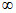# CAT – Inequalities

Question:
Solve the inequality x3 – 5x2 + 8x – 4 > 0.
A.  (2,)
B.  (1, 2) U (2,)
C.  (-, 1) U (2,)
D.  (-, 1)

Explanation:
Let a, b, c be the roots of this cubic equation
a + b + c = 5
ab + bc + ca = 8
abc = 4
This happens when a = 1, b = 2 and c = 2 {This is another approach to solving cubic equations}
The other approach is to use polynomial remainder theorem
If you notice, sum of the coefficients = 0
=>  P(1) = 0
=>  (x – 1) is a factor of the equation. Once we find one factor, we can find the other two by dividing the polynomial by (x – 1) and then factorizing the resulting quadratic equation.
(x – 1) (x – 2) (x – 2) > 0
Let us call the product (x – 1)(x – 2)(x – 2) as a black box.
If x is less than 1, the black box is a –ve number
If x is between 1 and 2, the black box is a +ve number
If x is greater than 2, the black box is a +ve number
Since we are searching for the regions where black box is a +ve number, the solution is as follows:
1 < x < 2 OR x > 2